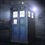# PLEASE HELP!!

Hello everybody!! I had my FTRE(FIITJEE TALENT REWARD EXAM) yesterday.This exam is held all over India and I think you all must have understood the purpose!There was this question that I have no idea how to solve and I was thinking if someone could help me.Anyway,the question is,$\overline{ab}\times \overline{c}=\overline{def}$.These conditions apply on the digits,$(a,b,c,d,e,f):\\ \bullet they\ are\ distinct\\ \bullet none\ of\ them\ is\ equal\ to\ 0\\ \bullet they\ are\ all\ less\ than\ 7.$Find the digits.Note by Adarsh Kumar
6 years, 5 months ago

This discussion board is a place to discuss our Daily Challenges and the math and science related to those challenges. Explanations are more than just a solution — they should explain the steps and thinking strategies that you used to obtain the solution. Comments should further the discussion of math and science.

When posting on Brilliant:

• Use the emojis to react to an explanation, whether you're congratulating a job well done , or just really confused .
• Ask specific questions about the challenge or the steps in somebody's explanation. Well-posed questions can add a lot to the discussion, but posting "I don't understand!" doesn't help anyone.
• Try to contribute something new to the discussion, whether it is an extension, generalization or other idea related to the challenge.
• Stay on topic — we're all here to learn more about math and science, not to hear about your favorite get-rich-quick scheme or current world events.

MarkdownAppears as
*italics* or _italics_ italics
**bold** or __bold__ bold
- bulleted- list
• bulleted
• list
1. numbered2. list
1. numbered
2. list
Note: you must add a full line of space before and after lists for them to show up correctly
paragraph 1paragraph 2

paragraph 1

paragraph 2

[example link](https://brilliant.org)example link
> This is a quote
This is a quote
    # I indented these lines
# 4 spaces, and now they show
# up as a code block.

print "hello world"
# I indented these lines
# 4 spaces, and now they show
# up as a code block.

print "hello world"
MathAppears as
Remember to wrap math in $$ ... $$ or $ ... $ to ensure proper formatting.
2 \times 3 $2 \times 3$
2^{34} $2^{34}$
a_{i-1} $a_{i-1}$
\frac{2}{3} $\frac{2}{3}$
\sqrt{2} $\sqrt{2}$
\sum_{i=1}^3 $\sum_{i=1}^3$
\sin \theta $\sin \theta$
\boxed{123} $\boxed{123}$

## Comments

Sort by:

Top Newest

$54\times 3=162$

- 6 years, 5 months ago

Log in to reply

awesome!Could you please tell how you got that.

- 6 years, 5 months ago

Log in to reply

• Firstly since all the digits are distinct so $b, c, f\not = 5$, moreover $d_{max}=3$ ( max occurs at $54\times 6=324$ )so only $a=5$ or $e=5$ .

• $b,c\not = 1$(as that would result in repetition)

• $f\not = 1$ (as 1 would occur only at $u(1\times 1)=1$, $u(7\times 3) =1$, $u(9\times 9)=1$ none of which satisfy the conditions.)

We start with $a=5$,

• If $b=2$ , then $c$ can be $3, 6$

• If $b=3$ , then $c$ can be $2, 4$

• If $b=4$ , then $c$ can be $3$

• If $b=6$ , then $c$ can be $2$

A quick check gives us the solution.

NOTE

• In the last step we cross out some values which give us a bigger unit's digit than 6 or if a repetition occurs.

• $u(x)$ means unit's digit of $x$.

- 6 years, 5 months ago

Log in to reply

Thanx dude!

- 6 years, 5 months ago

Log in to reply

54 *3 = 162

- 6 years, 5 months ago

Log in to reply

yup!that is correct!

- 6 years, 5 months ago

Log in to reply

See such questions could by setting few conditions by ourselves-

lets take it that way -- if b =x then c must be what such that f is not equal to c or b

If we put b as 1 then all values of f would be equal to c.

If we put b as 2 we see that c could be = 3

If we put b as 3 we see that c could be = 2 , 4

If we put b as 4 wee see that c could be = 3

5 can never stand on our suppositions

even 6 won't be

so we examine the 4 values of b = 2, 3 , 4 and c also 2 , 3 , 4

then we think for value of a

If a = 1 then it won't be 3 digit number . Similarly even 2 won' t be - Then further we could do trial & error wid the remaining values

hence , 54*3 = 162

- 6 years, 5 months ago

Log in to reply

54x3=162

- 6 years, 5 months ago

Log in to reply

thanku!

- 6 years, 5 months ago

Log in to reply

- 6 years, 5 months ago

Log in to reply

×

Problem Loading...

Note Loading...

Set Loading...